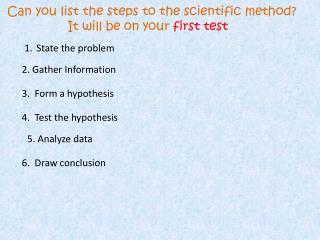DownloadDownload PresentationCan you list the steps to the scientific method? It will be on your first test

# Can you list the steps to the scientific method? It will be on your first test

Download Presentation## Can you list the steps to the scientific method? It will be on your first test

- - - - - - - - - - - - - - - - - - - - - - - - - - - E N D - - - - - - - - - - - - - - - - - - - - - - - - - - -
##### Presentation Transcript

1. Can you list the steps to the scientific method? It will be on your first test State the problem 2. Gather Information 3. Form a hypothesis 4. Test the hypothesis 5. Analyze data 6. Draw conclusion

2. What is missing ? What do you think of when you hear 32 degrees 32◦ ___ (SCALE) Fahrenheit, Celsius, Kelvin United States Scientist & majority other countries Measures VERY high Or VERY low temps. 32◦ Fahrenheit 32◦ Celsius = 89◦ Fahrenheit 273◦Kelvin

3. Chapter 1 Section 1.2Standards of Measurement

4. A. Standard- exact quantity that people agree to use for comparison B. Measurements must have a number and a unit .

5. 1. SI – (International system of standards) an improved version of the metric system used and understood by scientists worldwide. 2. SI system is based on multiples of 10 and uses prefixes to indicate a specific multiple.

6. Lengthis measured using a unit appropriate for the distance between two points. SI unit – meter (m) • Volumethe amount of space an object occupies. SI unit – liter (L)

7. Mass - measured of matter in an object. SI unit = kilogram (kg) What’s the difference between mass and weight? Mass = measure of matter (amount of “stuff” you are made up of)… your mass stays the same whether you are on Earth or the moon or Neptune Weight = mass + gravity pulling on that object …so you would weigh less on the moon since there is less gravity http://www.exploratorium.edu/ronh/weight/ Mass =Triple beam balance Weight Scale

8. What do you have to do with Italian salad dressing before using it? 1. Density - mass per unit volume of a material. 2. A unit obtained by combining different SI units is called a derived unit. Example: g/cm3 less dense

9. Time is the interval between two events; The SI unit for time is seconds (s) G. Temperature is measured using a thermometer. The SI unit of temperature is Kelvin (k)